### IMO Shortlist 2015 problem C5

Kvaliteta:
Avg: 0.0
Težina:
Avg: 8.0

The sequence$a_1,a_2,\dots$ of integers satisfies the conditions:

(i)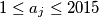$1\le a_j\le2015$ for all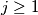$j\ge1$,
(ii)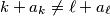$k+a_k\neq \ell+a_\ell$ for all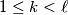$1\le k<\ell$.

Prove that there exist two positive integers$b$ and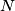$N$ for whichfor all integers$m$ and$n$ such that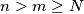$n>m\ge N$.

(Australia)

Source: https://www.imo-official.org/problems/IMO2015SL.pdf